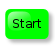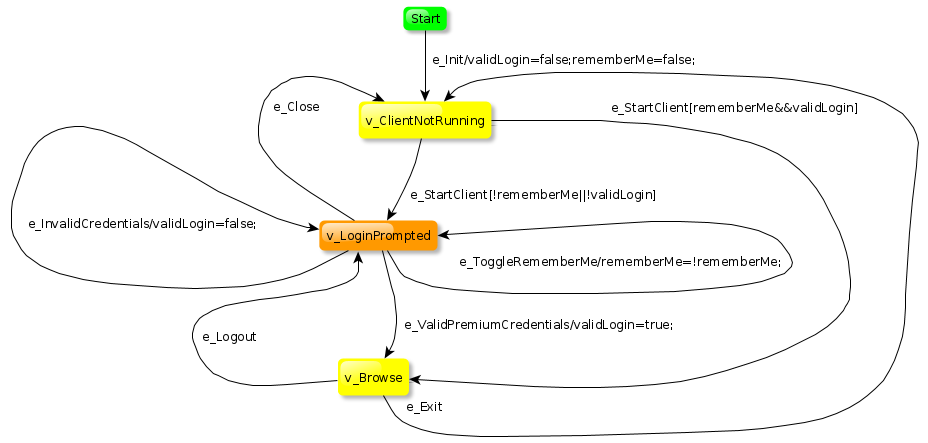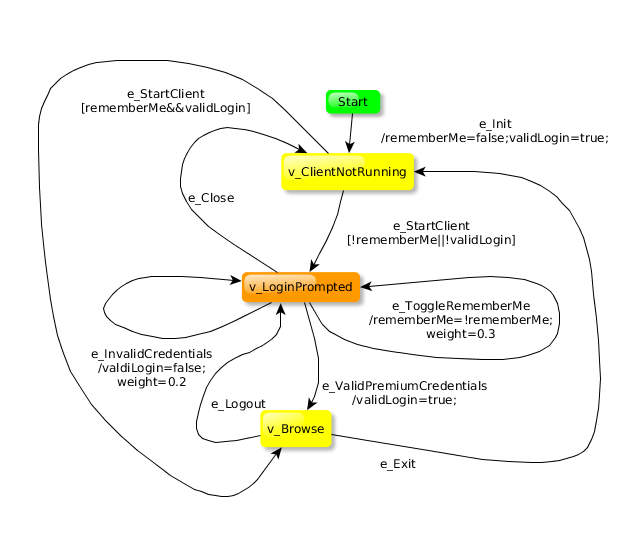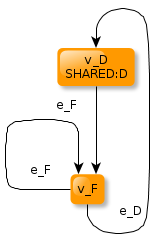GraphWalker is a open source Model-based testing tool for test automation. When modeling for GraphWalker using the yEd editor, there's a special syntax and rule set to follow, this pages explains how.

This describes the syntax for the GraphWalker, and what the rules are when a model is created using the yEd model editor.

## The model is a directed graph

The objective of the model, is to express the expected behavior of the system under test. To do so, we use a directed graph, in which a vertex (or a node) represents some desired state, and the edges (arcs, arrows, transitions) represents whatever actions we need to do in order to achieve that desired state.

For example, let’s take a web site that requires authentication before we can access the sites content. Designing a test, using a directed graph, might look like this:## Vertex

A vertex represents an expected state that we want to examine. In any implementing code/test, there is where you’ll find the assertions, or the oracles.

• In yEd a vertex is called node, normally depicted as a box.
• GraphWalker does not care what colors or shape a vertex has.

## Edge

Represents the transition from one vertex to another. It is whatever action is needed to be made in order to reach the next state. It could be selecting some menu choice, clicking a button, or making a REST API call.

• GraphWalker only accepts one-way directed edges (arrows).
• GraphWalker does not care what colors or thickness an edge has.

## The Rules

This section will talk about the modeling rules using yEd with GraphWalker.

### Start vertex• The Start vertex is not mandatory.
• If used, there must be 1 (and only 1) vertex with the name: Start in a model.
• There can only be 1 out-edge from the Start vertex.
• The Start vertex will not be included in any generated path.

### Name of a vertex or edge

The name is the first word, on the first line in a label for an edge or vertex.

### Label

A label is all the text associated to an edge or a vertex.

### Guards - Only for an edge

Guards are a mechanism only associated with edges. Their roles are the same as an if-statement, and makes an edge eligible or not for being walked.

The guard is a conditional expression enclosed between square brackets:

``````[loggedIn == true]
``````

The above means that if the attribute loggedIn equals to true, the edge is accessible.

### Action - Only for an edge

Action is a mechanism only associated to edges. This is javascript code that we want to execute in the model. It’s placed after a forward slash. Each statement must be ended with a semicolon.

``````/loggedIn=false; rememberMe=true;
``````

The purpose of the action code, is to serve as data to the guards.

#### ExampleThis example illustrates how actions and guards work.

1) Lets start with the out-edge from the Start vertex:

``````e_Init/validLogin=false;rememberMe=false;
``````

The name of the edge is e_Init, followed by a forward slash, denoting that text from that point until end-of-line is [action] code. The action initializes 2 attributes: validLogin and rememberMe.

2) When we have walked the edge above, we arrive at the v_ClientNotRunning vertex. This vertex has 2 out-edges, which both have guards. Since both validLogin and rememberMe are at this point initialized to false, only 1 edge is accessible for walking: the edge e_Start that has the vertex v_LoginPrompted as destination.

3) Now lets say that we have traversed the edges e_ToggleRememberMe and e_ValidPremiumCredentials and arrive again at the vertex v_ClientNotRunning, we would now expect GraphWalker to select the other e_Start that has the vertex v_Browse as destination.

This illustrates how we can direct and control flows through a graph, if we need to do that.

### Global attributes - Share data between models

In the examples above, where `loggedIn`, `validLogin` and `rememberMe` was used as attributes to hold data in a model, they cannot be shared between models.

To achive that, a global attribute is used instead. Like thís:

For an action:

``````/global.loggedIn=false; global.rememberMe=true;
``````

For a guard:

``````[global.loggedIn == true]
``````

### Keywords

Keywords are used in the models to increase functionality and usability.

• Start - This is used in a vertex to denote the Start vertex. Only one Start vertex per model.

• BLOCKED - A vertex or an edge containing this keyword, will be exclude when a path is generated. If it’s an edge, it will simply be removed from the graph. If it’s a vertex, the vertex will be removed with its in- and out-edges.

• SHARED - This keyword is only for vertices. It means that GraphWalker can jump out of the current model, to any other model to a vertex with the same SHARED name.
The syntax is:
SHARED:SOME_NAME

• INIT - Only a vertex can have this keyword. When using data in a model, the data needs to be initialized. That is what this keyword does. INIT is allowed in more vertices than just one.
The syntax is:
INIT:loggedIn=false; rememberMe=true;

• REQTAG - Only a vertex can have this keyword. A comma separated list of requirements. Used to create traceability with external requirements and the models.
The syntax is:
REQTAG:String1, String2

• weight - Only an edge can have this keyword. Used only when using the random generator. It holds a real value between 0 and 1, and represents the probability that the edge should be chosen. For example, a value of 0.05, would mean a 5% chance of that edge to be selected. The image below has 2 out-edges from vertex v_LoginPrompted that uses the keyword weight.
The syntax is:
weight=float value between 0.0 and 1.0### Multiple models

GraphWalker can work with several models in one session. It means that when generating a path, GraphWalker can choose to jump out of one model into another one. This is very handy when separating different functionality into several models. For example. Let’s say you have a system that you want to test, and you would need to log in to do that. Then it might make sense to create a single model handling the log in functionality, and other models to handle whatever else you want to test. The log in model would then be reused for ever other test scenario.

#### It’s not the same thing as flattening

When flatting models, several models are merged into one single model, which then is being traversed by GraphWalker. This is not the case here. GraphWalker is executing every model in its own context. The scope of the data in the models are not shared between them.

#### SHARED:SOME_NAME

The mechanism that controls the jumping between the models is the keyword SHARED. Let’s look at an example. Consider these 4 models:All models are loaded into GraphWalker, and the first model (Model A) is where the path generation is started. Using graphwalker-cli, the command line could look something like this:

``````gw3 offline -m src/test/resources/graphml/shared_state/Model_A.graphml "random(edge_coverage(100))" \
-m src/test/resources/graphml/shared_state/Model_B.graphml "random(edge_coverage(100))" \
-m src/test/resources/graphml/shared_state/Model_C.graphml "random(edge_coverage(100))" \
-m src/test/resources/graphml/shared_state/Model_D.graphml "random(edge_coverage(100))"
``````

When the path generation reaches the vertex v_B in Model A, it has to consider the keyword SHARED:B.. This tells GraphWalker to search all other models for the same keyword using the same name: B. In our case, there is only one, and it’s in Model B. Now GraphWalker makes a decision whether to jump out of Model A, into the vertex v_B in Model B, or to stay in Model A. This decision is based on random.

Also, if the path generation is executing in Model B, and it reaches the vertex v_B, GraphWalker can jump out of Model B, back to vertex v_B in Model A.

Tags: